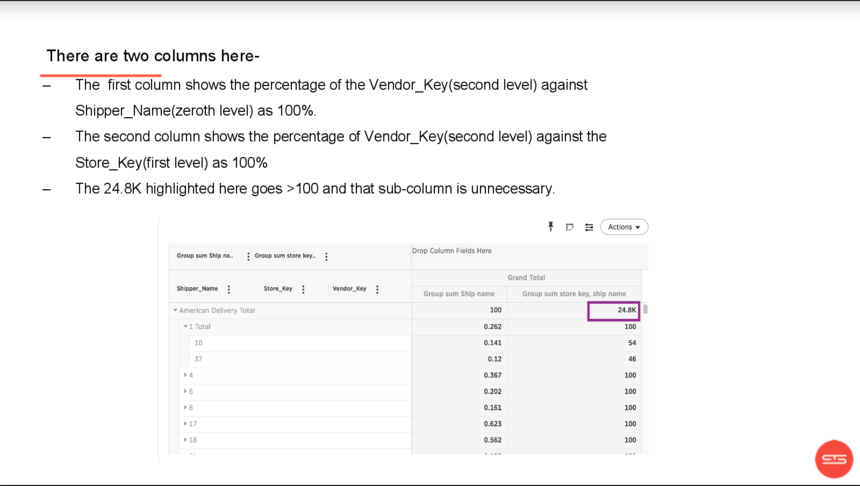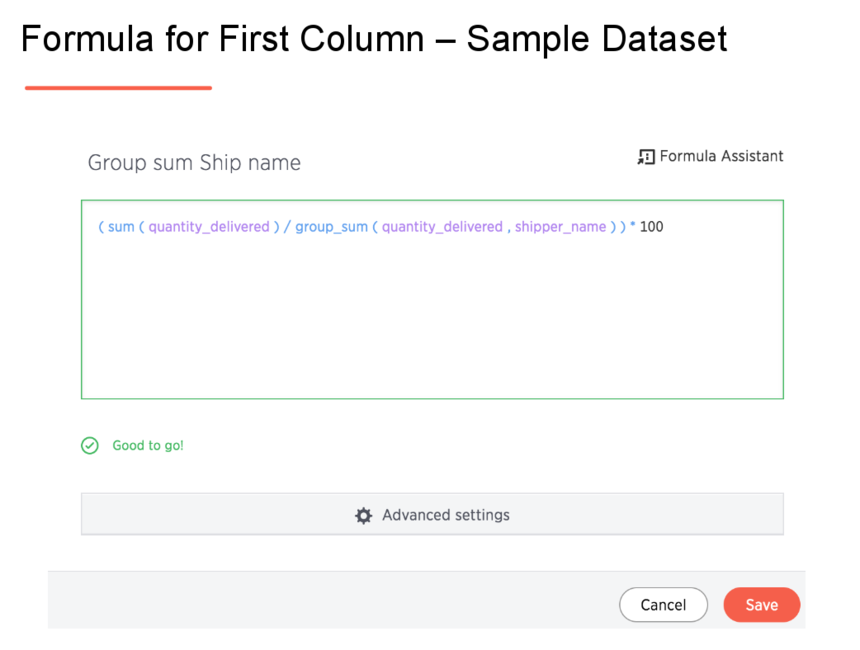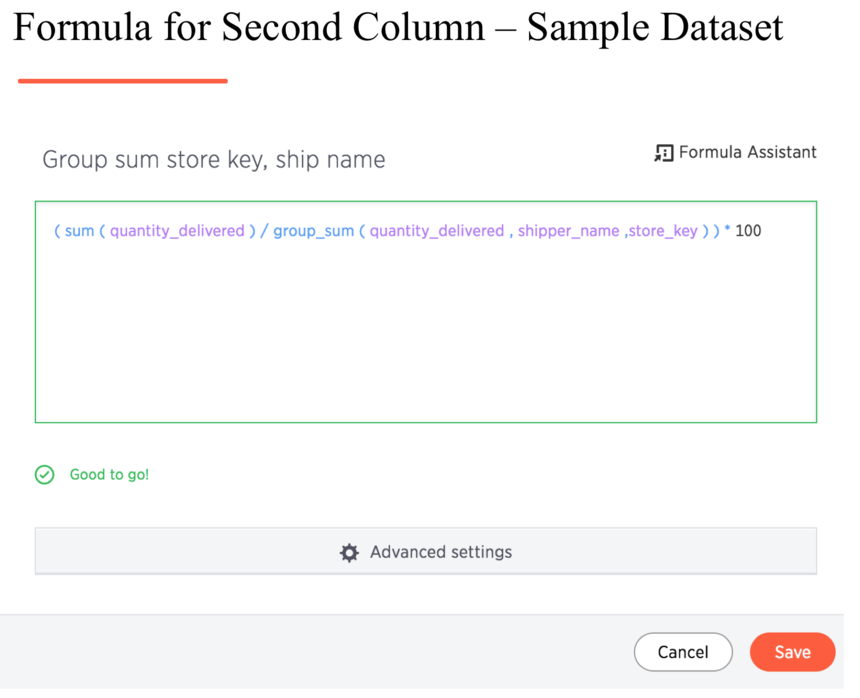# Calculate Percentage of Total

Hello,

I have a measure, PercentOfWeek, that I would like to view as a percentage of cumulative total(similar to Excel pivot table - Show values as % of Parent Row Total).  How can this be achieved?

Thanks!

3replies Oldest first
• Oldest first
• Popular•If I'm correctly understanding the question, you can use a formula, such as the following:  group_sum ( sales dollar amount , category description ) / group_sum ( sales dollar amount )

This will give you the % of total sales for a particular product category.

Like
•I would like results to view as example below.  The desired display is the percentage.  The value in parenthesis is how the data is currently displayed.

All projects nested under each employee need to be a percentage (adding to 100%)  In this example, I have four employees so each of their hours represent 25% of the Category Total.

Week Ending 11/17

Category Total                                          100% (4)

> Employee 1 Total                                  25% (1)

> Project 1                                            80% (.8)

> Project 2                                           20%(.2)

> Employee 2 Total                                 25% (1)

> Employee 3 Total                                 25% (1)

> Employee 4 Total                                 25% (1)

> Project 1                                             40% (.4)

> Project 2                                            50% (.5)

> Project 3                                            10% (.1)

Like
•Hi Paul,

Posting the solution I communicated to you via email. The requirement was to get the subsequent levels in a Pivot table as the percent of the parent row total. I have used two formula columns to achieve this.

Note: this is a sample datasetLike
Like Follow